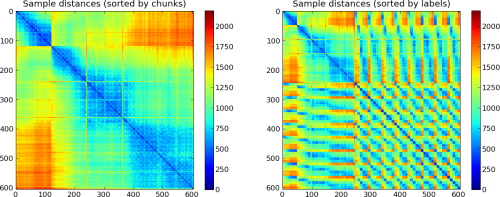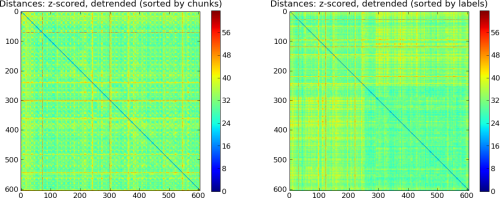# Simple Data-Exploration¶

Example showing some possibilities of data exploration (i.e. to ‘smell’ data).

```from mvpa2.suite import *

# only use the first 5 chunks to save some cpu-cycles
ds = ds[ds.chunks < 5]
```

It is always useful to have a quick look at the summary of the dataset and verify that statistics (mean, standard deviation) are in the expected range, that there is balance among targets/chunks, and that order is balanced (where appropriate).

```print ds.summary()
```

Now we can take a look at the distribution of the feature values in all sample categories and chunks.

```pl.figure(figsize=(14, 14)) # larger figure
hist(ds, xgroup_attr='chunks', ygroup_attr='targets', noticks=None,
bins=20, normed=True)

# next only works with floating point data
ds.samples = ds.samples.astype('float')

# look at sample similarity
# Note, the decreasing similarity with increasing temporal distance
# of the samples
pl.figure(figsize=(14, 6))
pl.subplot(121)
plot_samples_distance(ds, sortbyattr='chunks')
pl.title('Sample distances (sorted by chunks)')

# similar distance plot, but now samples sorted by their
# respective targets, i.e. samples with same targets are plotted
# Note, that the first and largest group corresponds to the
# 'rest' condition in the dataset
pl.subplot(122)
plot_samples_distance(ds, sortbyattr='targets')
pl.title('Sample distances (sorted by targets)')

# z-score features individually per chunk
print 'Detrending data'
poly_detrend(ds, polyord=2, chunks_attr='chunks')
print 'Z-Scoring data'
zscore(ds)

pl.figure(figsize=(14, 6))
pl.subplot(121)
plot_samples_distance(ds, sortbyattr='chunks')
pl.title('Distances: z-scored, detrended (sorted by chunks)')
pl.subplot(122)
plot_samples_distance(ds, sortbyattr='targets')
pl.title('Distances: z-scored, detrended (sorted by targets)');
# XXX add some more, maybe show effect of preprocessing
```

Outputs of the example script. Data prior to preprocessingData after minimal preprocessingThe full source code of this example is included in the PyMVPA source distribution (`doc/examples/smellit.py`).